# Why isn't this Op Amp saturated ?

Status
Not open for further replies.

#### Xenon02

Joined Feb 24, 2021
355
Hello !

I've got a problem because I don't know either if it's my fault or is it simulator fault. But when I give positive voltage into negative input of the Op amp the output is also positive ? I've seen some picture that says that when I give positive voltage into negative input of the Op Amp then I have negative output.

My circuit :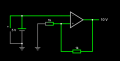And the picture I was talking about :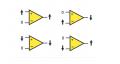So how does it work ? In the picture it shows that when I give positive voltage into negative input then I have negative output.

#### ericgibbs

Joined Jan 29, 2010
17,150
hi,
E

#### Attachments

• 116.1 KB Views: 12

#### BobTPH

Joined Jun 5, 2013
6,296
You have positive feedback. That is not a stable configuration. Op amps need to have negative feedback.

•panic mode and dcbingaman

#### Xenon02

Joined Feb 24, 2021
355

#### crutschow

Joined Mar 14, 2008
31,511
So why I have positive on the output when I have positive on the negative input ?
It's not just the voltage on the negative input that determines the output.
The input is differential so it responds to the voltage difference between the two inputs.

You are right at the comparator trip point since the two resistors generate +5V at the positive input from the +10V output..
Try 5.1V at the negative input.

•dcbingaman

#### Xenon02

Joined Feb 24, 2021
355
It's not just the voltage on the negative input that determines the output.
The input is differential so it responds to the voltage difference between the two inputs.

You are right at the comparator trip point since the two resistors generate +5V at the positive input from the +10V output..
Try 5.1V at the negative input.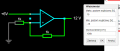So the simulator is right ?

#### MrChips

Joined Oct 2, 2009
28,127
Pay attention to feedback.
There is positive feedback and there is negative feedback.

Positive feedback is self reinforcing and leads to a runaway situation or saturation in an amplifier.
Negative feedback is self correcting and have a stabilizing effect.

With positive feedback, you are trying to balance a needle on its point. It can flip one way or the other.
What you have is a voltage comparator. The output flips state depending (+IN) - (-IN)

Know this rule
With proper negative feedback, the voltage on the inverting input is the same as that on the non-inverting input.
In other words, the output tries to make the voltage difference between the inverting and non-inverting inputs zero, i.e. (+IN) - (-IN) = 0.

•panic mode

#### Xenon02

Joined Feb 24, 2021
355
Also you said that not only the input determines the output so why when I have this circuit :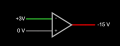I have negative on the output when I give positive on the negative input? Like in this picture :But it doesn't work in my first circuit ?

#### Wendy

Joined Mar 24, 2008
23,126
Always remember, the input of the opamp or comparator must be connected to something, be it ground or a voltage.
Did you read my post # 5?

#### Xenon02

Joined Feb 24, 2021
355
Pay attention to feedback.
There is positive feedback and there is negative feedback.

Positive feedback is self reinforcing and leads to a runaway situation or saturation in an amplifier.
Negative feedback is self correcting and have a stabilizing effect.

Know this rule
With proper negative feedback, the voltage on the inverting input is the same as that on the non-inverting input.
In other words, the output tries to make the voltage difference between the inverting and non-inverting inputs zero.
So the simulation is right ?
Why in some websites they say that when i give positive voltage to a negative input then I have negative output. Like the picture I have posted, and the simulations shows the same but when I give positive feedback then when I give positive voltage to a negative input then I have a positive output. Why is it like that ?

#### MrChips

Joined Oct 2, 2009
28,127
So the simulation is right ?
Why in some websites they say that when i give positive voltage to a negative input then I have negative output. Like the picture I have posted, and the simulations shows the same but when I give positive feedback then when I give positive voltage to a negative input then I have a positive output. Why is it like that ?
Those statements are incorrect.
It is not the polarity that matters. It is the voltage difference that matters.

(+IN) - (-IN)

#### crutschow

Joined Mar 14, 2008
31,511
Why is it like that ?
You simple simulator shows a varying saturation voltage with input.
A real op will be saturated at close to the supply rail voltages.
How is the maximum op amp output voltage determined on your simulator?

You may need a better simulator, such as the free LTspice from Analog Devices

#### BobTPH

Joined Jun 5, 2013
6,296
A Schmidt trigger has two thresholds. If you give it a voltage between the two, the simulator cannot determine the correct output.

Look at the voltages in your first example. The voltage on both inputs is 5V. So, what should the output be?

#### MrChips

Joined Oct 2, 2009
28,127This is a voltage comparator.
What are the tipping points? In other words, what are the input threshold voltages?
You need to state the power supply voltages.

For example, if your supply voltages are +10V and 0V, your two tipping points are +5V and 0V.
If your supply voltages are +10V and -10V, your two tipping points are +5V and -5V.

(Take into account that a real op-amp cannot output the rail-rail voltages.)

#### crutschow

Joined Mar 14, 2008
31,511
(Take into account that a real op-amp cannot output the rail-rail voltages.)
Some get pretty close.

#### Xenon02

Joined Feb 24, 2021
355
Wait a second I need to know something.

You simple simulator shows a varying saturation voltage with input.
A real op will be saturated at close to the supply rail voltages.
How is the maximum op amp output voltage determined on your simulator?

You may need a better simulator, such as the free LTspice from Analog Devices
I've shown the screen what is the maximum voltage that is +15V and -15V.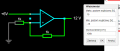But I know it is a different language so I'm sorry. I didn't to translate it.

So I don't know if the simulation is correct or not. Just a simple question yes or no.

A Schmidt trigger has two thresholds. If you give it a voltage between the two, the simulator cannot determine the correct output.

Look at the voltages in your first example. The voltage on both inputs is 5V. So, what should the output be?
Well I have only one voltage supply that is connected to the negative input. and the positive input is undetermined. As I think so.

View attachment 272626

This is a voltage comparator.
What are the tipping points? In other words, what are the input threshold voltages?
You need to state the power supply voltages.

For example, if your supply voltages are +10V and 0V, your two tipping points are +5V and 0V.
If your supply voltages are +10V and -10V, your two tipping points are +5V and -5V.

(Take into account that a real op-amp cannot output the rail-rail voltages.)
Tipping points ? You mean 2 supply voltage ? One is 10V and second one is 0 V ? Like 10V on negative input and 0V on the positive input ?

I don't know whether the simulator is right or not. Also you said that the input doesn't determine the output :

Those statements are incorrect.
It is not the polarity that matters. It is the voltage difference that matters.

(+IN) - (-IN)
So why here the output is negative ?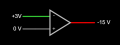If the polarity doesn't matter (of the inputs) then why the output is negative ? Of course this picture shows the saturation.

#### Papabravo

Joined Feb 24, 2006
19,846
...
Edit: What you are wanting is a comparator, AKA single byte A/D converter.
...
I think you meant single bit A/D converter.Mod edit: sheesh, everyone is a critic. Wendy

Status
Not open for further replies.